Up | CircleFinder2D - 165 | EasyOCR - 78 | PolygonMatch2 - 84 | GCPS -110 | HeightMap3D - 111 | ImageExtrema - 129 | PCA3D - 140 | CameraPose3D - 148 | MonoPose3D - 149 | ImagePicker3D - 145 | ExternalCoordinates3D - 157 | MaMa3D - 152 | CircleSegmentor - 153 | PolygonMatch3 - 160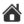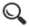MaMa3D - 152
This tool locates multiple objects in a point cloud using 3D Manifold Matching.

This tool needs an ordered point cloud.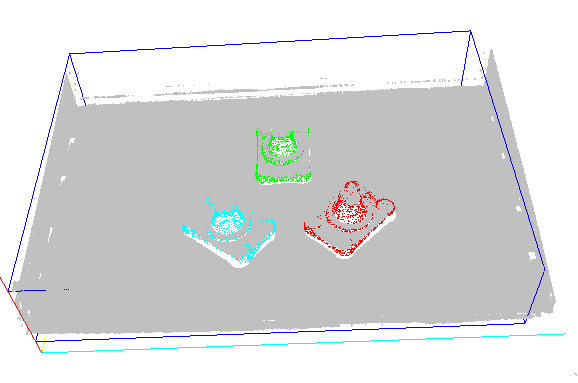Test profile

Here is a test profile

for the MaMa3D tool. It contains an ordered point cloud and a template object (flens.txt). With the existing setup the tool will find three objects in the point cloud using the supplied template. You can also experiment with the Import ROI to pick templates from the point cloud.

Setup

3D coordinate system

• Reference - select a 3D reference

Search parameters

Parameters that describe tolerances for matching reference point and search point in scene:
• Reference plane sizeDistance tolerance for reference point in reference point's tangent plane
• Search plane size - Distance tolerance for search point in search point's tangent plane
• Distance uncertaintyGeneral distance tolerance
• Angle uncertainty (deg)General angular tolerance
Parameters that control surface normal calculation:
• Min distance min z value of scene
• Max distance - max z value of scene
• Stride decimation factor of scene when calculating surface normals
• Normal size size of neighbourhood used to calculate surface normals (unit: pixels)
Parameters that control matching process:
• Epsilondistance tolerance used to check whether a template point is close enough to a scene point
• Min coverage (%) - percentage of visible template points that must be matched to a scene point
• Kill radiusminimum distance between found matches in scene

Point cloud filter

• Contrast - enabled/disabled
• Min/Max - element 4 in a 5-element point cloud; set min and max allowable value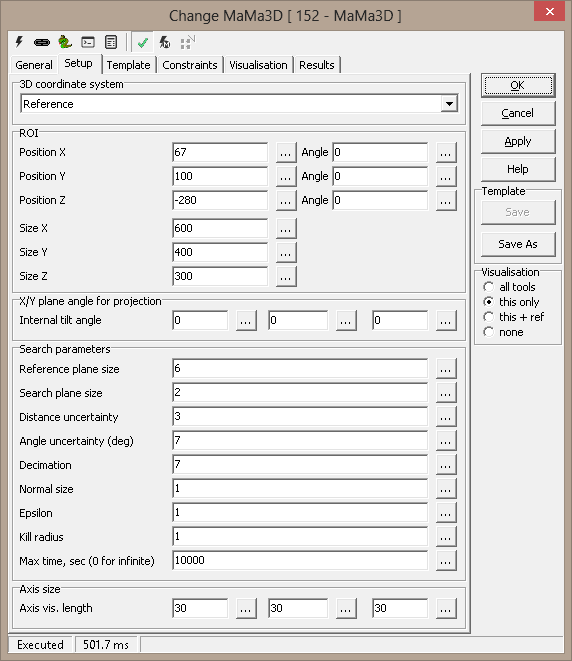Template

Template

This displays the model with axes, and the plane normal for the search plane. The reference system is defined from the reference and search planes.

• Reference plane/Search plane - lets you easily define these planes for the model:
• Click a dot in the model view
• Press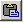(paste) for either the reference or search plane
• If needed, edit the x/y/z numbers and press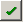to select the nearest point in the model
• Plane size - the size (radius) used to calculate the plane normals for the model, when reference or search points are set.
• Import from import ROI - see Import ROI (below)
• Load from file... - currently, only text files with a point position/plane normal (6 numbers) on each line are supported. If the file is considered normalized, the correct reference plane and search plane are implicitly set when loading the model.
• Save raw to file... - save current raw (original) model
• Save to file... - save normalized model. This saves the model with a normalized coordinate system, so that the reference point is defined as the origin (0,0,0), and the axes are oriented with x perpendicular to the reference plane, and y pointing towards the search plane.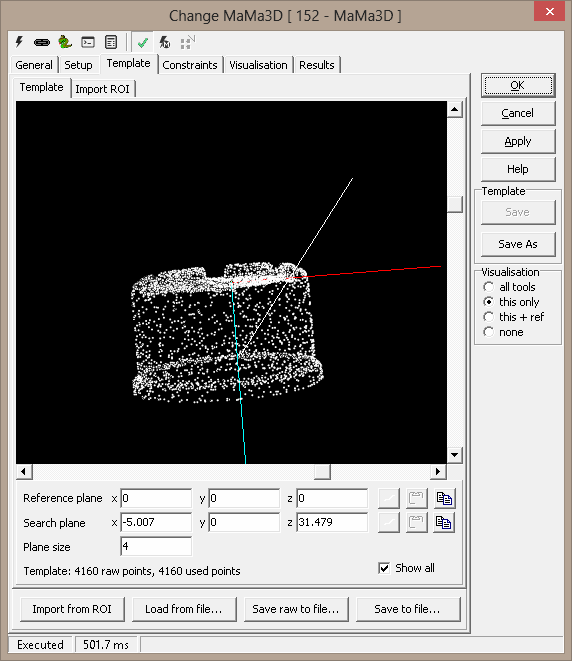Import ROI

Specify an ROI as either a box (cuboid), a cylinder segment or a sphere to extract a subsection from the current image as the template model.

• Position (X,Y,Z) - ROI center
• Angle (X,Y,Z) - ROI rotation
• Size (X,Y,Z) - Cuboid (box) size
• Length - Cylinder length
• Inner/Outer radius - Cylinder sector inner/outer radius
• Cylinder sector Start/End - Cylinder sector angles
• Point cloud decimation (x & y)
• Factor - decimation of point cloud
• Point cloud filter
• Contrast - Activate/deactivate
• Min/Max - contrast limits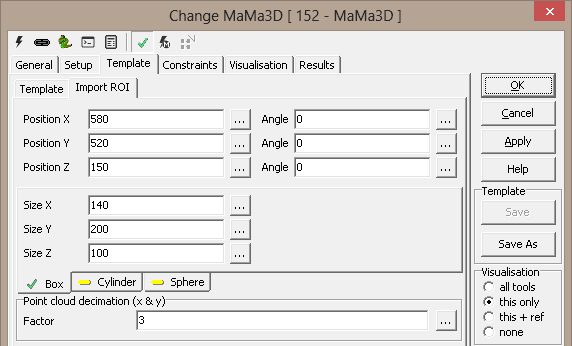Constraints

(none yet defined)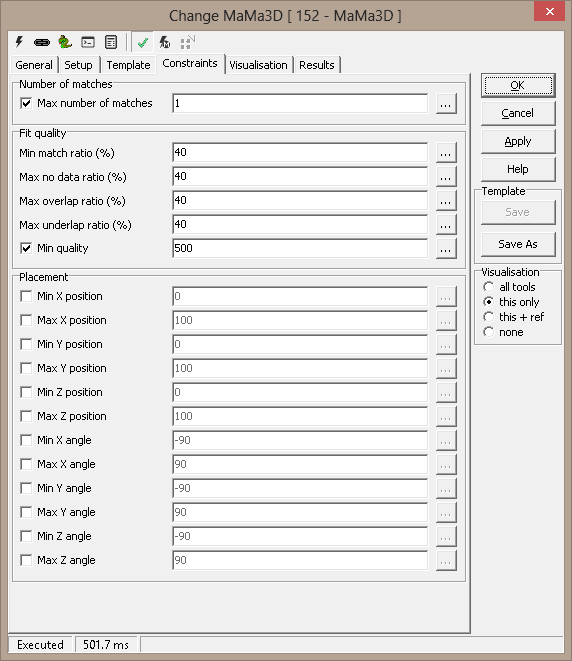Visualisation
 Match-Match The matched object(s)

Results

 Match count Number of found objects Initial template points Points in template model Used template points "Good" i.e. used points in template model Quality[n] Match result, object n. Smaller is better Hit percentage[n] Percentage of points with match, object n Position[n].x/.y/.z Position, object n Angle[n].x/.y/.z Rotation, object n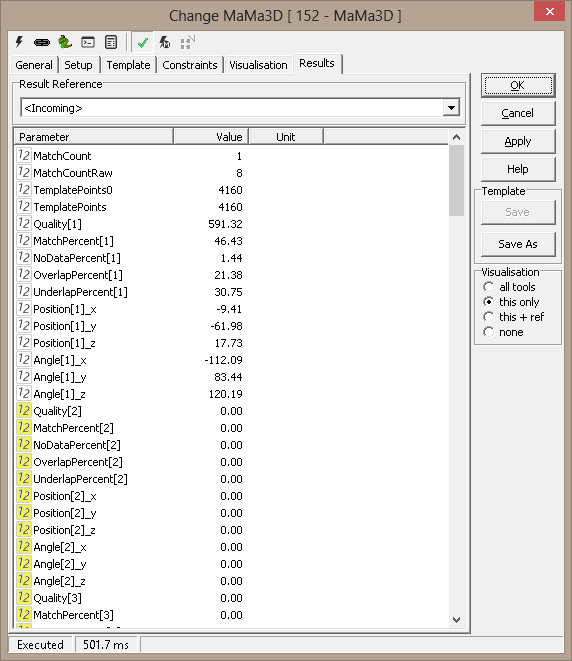ExecuteCmd support